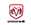•••###Sprinter Rear Axles

Some available Rear Axle options for the sprinter are:

Year:

• 5.10 ratio
• 3.92 ratio
• 4.72 ratio
• 4.10 ratio

• Year:

• 4.72 ratio
• 5.10 ratio
• 3.92 ratio
• 4.10 ratio

• Year:

• 3.92 ratio
• 5.10 ratio
• 4.72 ratio
• 4.10 ratio

• Year:

## 2006 dodge sprinter Rear Axle

• ABS 4.11 ratio
• w/o ABS 4.11 ratio
• w/o ABS 3.72 ratio
• ABS 3.72 ratio

• Year:

## 2005 dodge sprinter Rear Axle

• ABS 3.72 ratio
• ABS 4.11 ratio
• w/o ABS 3.72 ratio
• w/o ABS 4.11 ratio

• Year:

## 2004 dodge sprinter Rear Axle

• w/o ABS 3.72 ratio
• w/o ABS 4.11 ratio
• ABS 4.11 ratio
• ABS 3.72 ratio

• Year:

## 2003 dodge sprinter Rear Axle

• ABS 4.11 ratio
• w/o ABS 3.72 ratio
• w/o ABS 4.11 ratio
• ABS 3.72 ratio

• Year:

## 2002 dodge sprinter Rear Axle

• ABS 3.72 ratio
• ABS 4.11 ratio
• w/o ABS 4.11 ratio
• w/o ABS 3.72 ratio